## Calculation of the Radius of Gyration (Rg) of the Truncated Icosahedron and of the Rhombicuboctahedron

As an example, the Radius of Gyration (Rg) of a Truncated Icosahedron (the shape of the C60-molecule) is being calculated. The polyhedral shape consists of 20 hexagons and 12 pentagons (60 vertices, 32 faces, 90 edges) with an unilateral edge-length a (here we take unit length = 1). All parameters for the calculation of Rg, such as out-, mid- and in-sphere radii (ro, rm, ri), respectively, can be found at Wolfram MathWorld .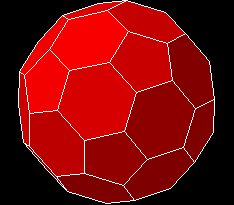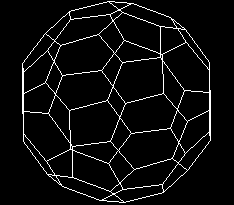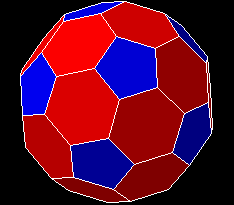The parameters for the solid shape are entered as input into the Rg-calculator as shown below: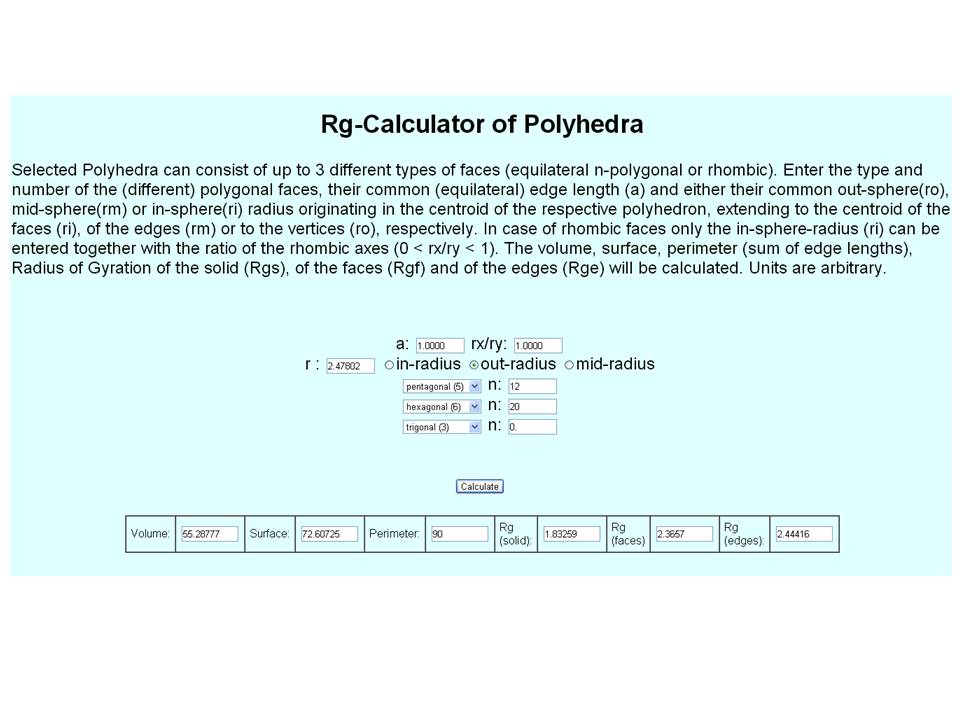Parameter (Truncated Icosahedron) Value Volume 55.288 Surface 72.607 Perimeter 90.000 out-sphere radius ro 2.478 mid-sphere radius rm 2.427 in-sphere radius ri (pentagons) 2.327 in-sphere radius ri (hexagons) 2.267 Rg of solid 1.833 Rg of faces 2.366 Rg of edges 2.444 Rg of vertices ( = ro) 2.478

Table 1. Shape parameters and calculated values for Rg (rounded) of the regular truncated icosahedron (C60) of unit length a=1 ( = edge length). Rg scales linearly with the edge-length a. Units are arbitrary.As another example, the Radius of Gyration (Rg) of the Small Rhombicuboctahedron (RCO) and of two of its variations (Small Cubicuboctahedron (CCO) and Small Rhombihexahedron (RH)) are being calculated. The polyhedral shape consists of 8 trigons and 18 tetragons (24 vertices, 26 faces, 48 edges) with an unilateral edge-length a (here we take unit length = 1). All parameters for the calculation of Rg, such as out-, mid- and in-sphere radii (ro, rm, ri), respectively, can be found at Wolfram MathWorld .

The parameters for the solid shape are entered as input into the Rg-calculator as shown below: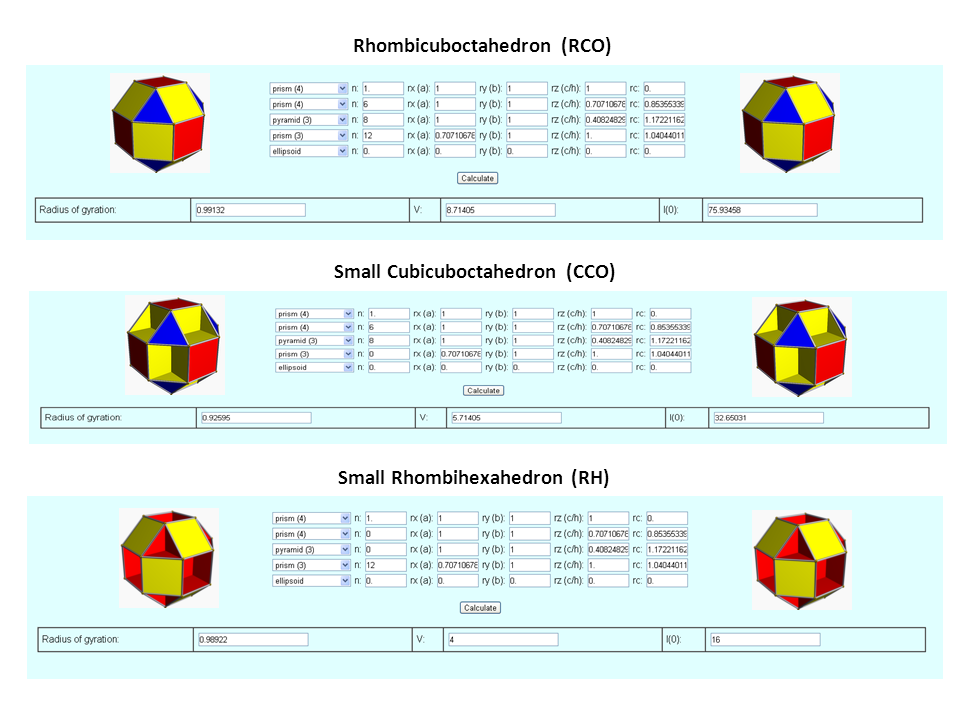Parameter (Small Rhombicuboctahedron) Value Volume 8.714 Surface 21.464 Perimeter 48.000 out-sphere radius ro 1.399 mid-sphere radius rm 1.307 in-sphere radius ri (trigons) 1.274 in-sphere radius ri (tetragons) 1.207 Rg (RCO) 0.991 Rg (CCO) 0.926 Rg (RH) 0.989

Table 2. Shape parameters and calculated values for Rg (rounded) of the Samll Rhombicuboctahedron of unit length a=1 ( = edge length). Rg scales linearly with the edge-length a. Units are arbitrary.Author: M.Kriechbaum, TU-Graz (2013), e-mail: manfred.kriechbaum@tugraz.at